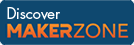Cody

# Problem 5. Triangle Numbers

Created by Cody Team in Community

Triangle numbers are the sums of successive integers. So 6 is a triangle number because

` 6 = 1 + 2 + 3`

which can be displayed in a triangular shape like so

```      *
* *
* * * ```

Thus 6 = triangle(3). Given n, return t, the triangular number for n.

Example:

``` Input  n = 4
Output t is 10```

### Solution Stats

64.37% Correct | 35.63% Incorrect
Last solution submitted on Mar 19, 2019

#### TagsMATLAB and Simulink resources for Arduino, LEGO, and Raspberry Pi